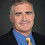## Thursday, May 25, 2023

### Finding Primes Pseudodeterministically

In 2003, Agrawal, Kayal and Saxena showed that primality testing is in P, i.e., you could test for primes without using any randomness.

What if you want to find a prime? Specifically given a number m in binary, can you find a prime p > m in time polynomial in the length of m. In 2009 I posted about a polymath project to find a deterministic algorithm for finding primes and the problem remains open today.

Likely you could just try m+1,m+2, ... until you find a prime but whether that is bounded by a polynomial number of steps is a big open question in number theory. You can choose random numbers between m and 2m and find one in expected polytime given the prime number theorem. This algorithm will likely output a different prime every time you run it.

There's a new paper by Lijie Chen, Zhenjian Lu, Igor Carboni Oliveira, Hanlin Ren and Rahul Santhanam that solves this problem pseudodeterministically for infinitely many inputs. This is a randomized polynomial-time algorithm B that for infinitely many n, there is a specific prime p between 2n and 2n+1 such that B(1n) outputs p with high probability. With high probability you will get the same prime every time you run the algorithm!

The proof uses a win-win kind of argument, if a certain algorithm fails to work you can use that to derandomize. Making that argument work requires bootstrapping on variants of previous pseudorandom generator and hitting sets constructions.

Almost surely there is a deterministic polytime algorithm for finding primes. But for now the new result of Chen et al. is a nice stop in that direction.

1.It seems that if the algorithm just outputs B(m) = m always, this should also work infinitely often (namely, when m is a prime.) The algorithm can also check if m is a prime in deterministic polynomial time.

Could you explain what properties this new algorithm has that the trivial algorithm does not have?

1.Good point. I made it trivial as written. The paper really says for infinitely many n, B(1^n) will output a prime of length n. I'll fix the post.

2.1^n is always 1

3.In this context 1^n is the string of n one's. It's a way to use n as an input and have B run in time polynomial in n.

2.What is the runtime of

if (n<2) return false;

stop = sqrt(n);

for i = 2 to stop if (n%i == 0) return false;

return true;

1.That takes sqrt(n) time. Remember though that when we talk about the complexity of computing primes or factoring, the length of the input is the length of the number written in binary, in this case log n. So this algorithm is exponential in its length.